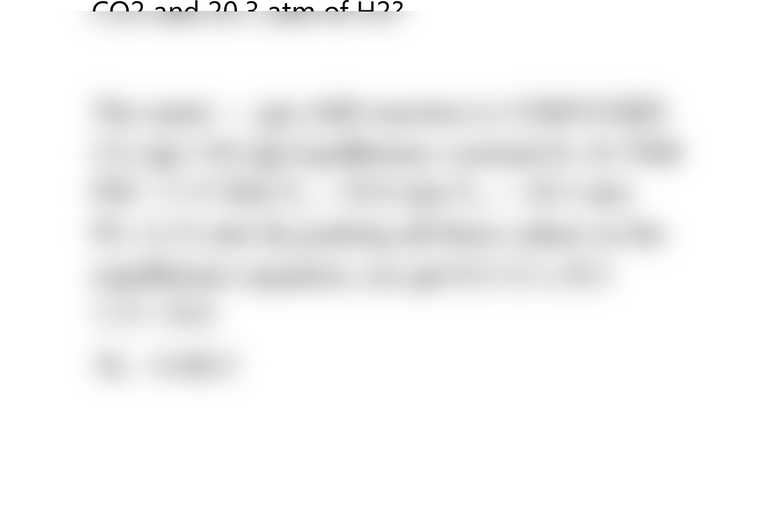# CAD-8104 Lecture Notes - Lecture 10: Water-Gas Shift Reaction, Equilibrium Constant, Chemical Equation

7 views2 pages
School
Department
Course
ProfessorCHEM 2426 HOMEWORK SOLUTIONS
In the industrial synthesis of hydrogen, mixtures
of CO andH2,am enriched inH2,by allowing the
CO to react with steam. The chemical equation
for this so-called water-gas shift reaction is
CO(g) + H20(g) c02(g) + H2(g)
What is the value ofKvat 700 K if the partial
pressures in an equilibrium mixture at 700 K are
1.31 atm of CO, 10.0 atm of H202 6.12 atm of
CO2 and 20.3 atm of H2?
The water gas shift reaction is CO(04120(00
CO, (g)+142 (g) Equilibrium constant K, At 700K
P00 =1.31 Wm P„, =10.0 atm P„, = 20.3 atm
P0,=6.12 atm By putting all these values in the
equilibrium equation, we get K 6.12 x 20.3
1.31=10.0
1K, =9.4831
Unlock document

This preview shows half of the first page of the document.
Unlock all 2 pages and 3 million more documents.